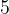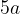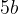## Saturday, 9 August 2014

### chapter 2 Quadrilaterals and Parallelograms

Theorem$5$
The opposite sides and opposite interior angles of a parallelogram are equal.Theorem$5a$
If a quadrilateral has opposite sides equal then it is a parallelogram.Theorem$5b$
If a quadrilateral has opposite interior angles equal then it is a parallelogram.Theorem$6$
The diagonals of a parallelogram bisect each other.Theorem$7$
The diagonals of a rhombus are perpendicular.